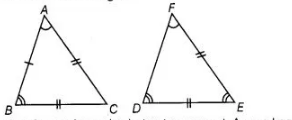# In ΔABC and ΔPQR, if AB = AC,`
Question:

In ΔABC and ΔPQR, if AB = AC, ∠C =∠P and ∠B = ∠Q, then the two triangles are

(a) isosceles but not congruent

(b) isosceles and congruent

(c) congruent but not isosceles

(d) Neither congruent nor isosceles

Solution:

(a) $\ln \triangle A B C$, $A B=A C$ [given]

$\Rightarrow \quad \angle C=\angle B \quad$ [angles opposite to equal sides are equal]

So, $\triangle A B C$ is an isosceles triangle.

But it is given that, $\angle B=\angle Q$

$\angle C=\angle P$

$\therefore \quad \angle P=\angle Q \quad[\because \angle C=\angle B]$

$\Rightarrow \quad Q R=P R \quad$ [sides opposite to equal angles are equal]

So, $\triangle P Q R$ is also an isosceles triangle.Therefore, both triangles are isosceles but not congruent. As, we know that AAA is not a criterion for congruence of triangles.